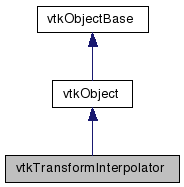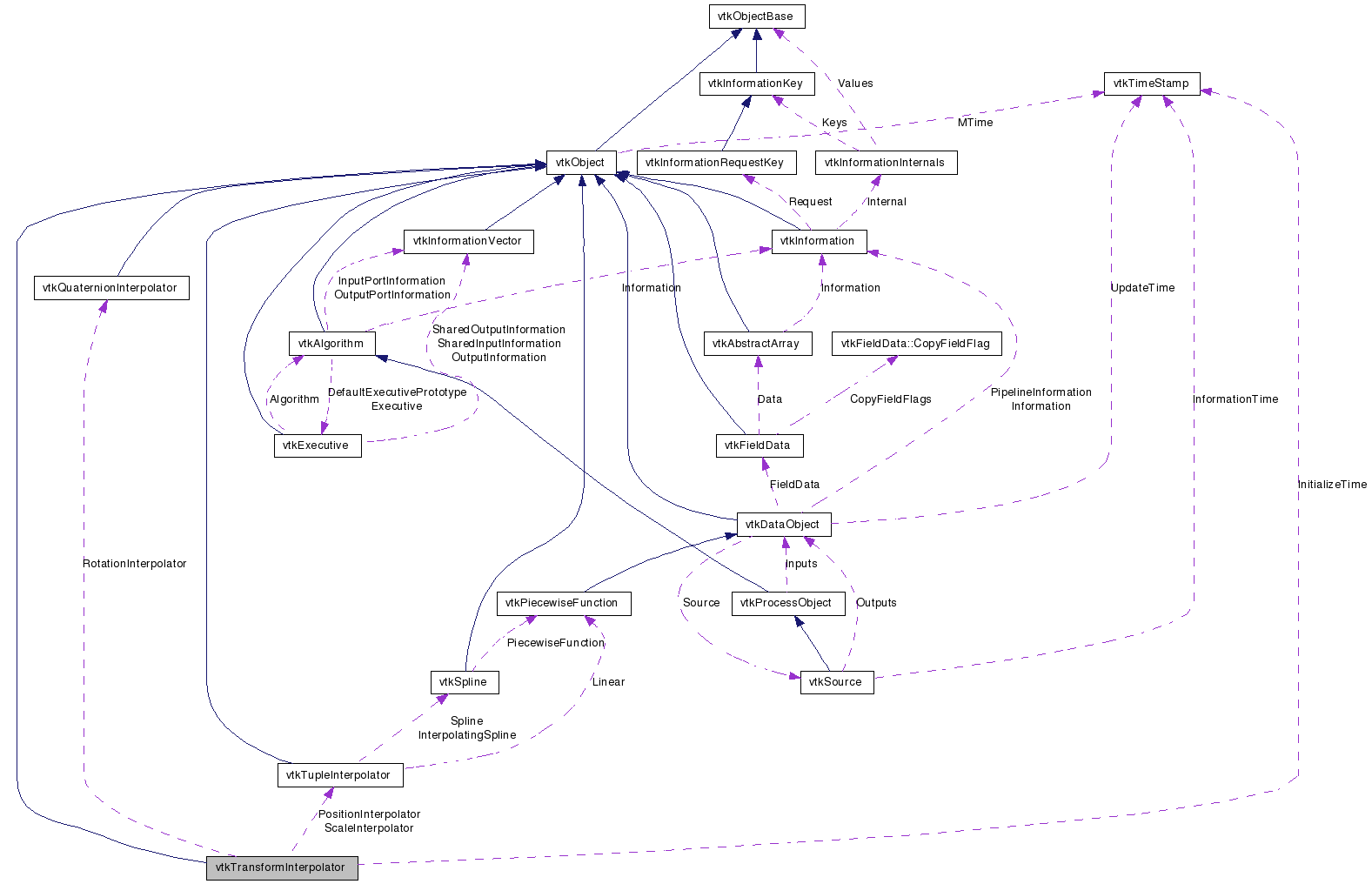# vtkTransformInterpolator Class Reference

`#include <vtkTransformInterpolator.h>`

Inheritance diagram for vtkTransformInterpolator:[legend]
Collaboration diagram for vtkTransformInterpolator:[legend]

## Detailed Description

interpolate a series of transformation matrices

This class is used to interpolate a series of 4x4 transformation matrices. Position, scale and orientation (i.e., rotations) are interpolated separately, and can be interpolated linearly or with a spline function. Note that orientation is interpolated using quaternions via SLERP (spherical linear interpolation) or the special vtkQuaternionSpline class.

To use this class, specify at least two pairs of (t,transformation matrix) with the AddTransform() method. Then interpolated the transforms with the InterpolateTransform(t,transform) method, where "t" must be in the range of (min,max) times specified by the AddTransform() method.

By default, spline interpolation is used for the interpolation of the transformation matrices. The position, scale and orientation of the matrices are interpolated with instances of the classes vtkTupleInterpolator (position,scale) and vtkQuaternionInterpolator (rotation). The user can override the interpolation behavior by gaining access to these separate interpolation classes. These interpolator classes (vtkTupleInterpolator and vtkQuaternionInterpolator) can be modified to perform linear versus spline interpolation, and/or different spline basis functions can be specified.

Warning:
The interpolator classes are initialized when the InterpolateTransform() is called. Any changes to the interpolators, or additions to the list of transforms to be interpolated, causes a reinitialization of the interpolators the next time InterpolateTransform() is invoked. Thus the best performance is obtained by 1) configuring the interpolators, 2) adding all the transforms, and 3) finally performing interpolation.
Tests:
vtkTransformInterpolator (Tests)

Definition at line 66 of file vtkTransformInterpolator.h.

## Public Types

typedef vtkObject Superclass
enum  { INTERPOLATION_TYPE_LINEAR = 0, INTERPOLATION_TYPE_SPLINE, INTERPOLATION_TYPE_MANUAL }

## Public Member Functions

virtual const char * GetClassName ()
virtual int IsA (const char *type)
void PrintSelf (ostream &os, vtkIndent indent)
int GetNumberOfTransforms ()
void Initialize ()
void RemoveTransform (double t)
void InterpolateTransform (double t, vtkTransform *xform)
unsigned long GetMTime ()
double GetMinimumT ()
double GetMaximumT ()
void AddTransform (double t, vtkTransform *xform)
void AddTransform (double t, vtkMatrix4x4 *matrix)
void AddTransform (double t, vtkProp3D *prop3D)
virtual void SetInterpolationType (int)
virtual int GetInterpolationType ()
void SetInterpolationTypeToLinear ()
void SetInterpolationTypeToSpline ()
void SetInterpolationTypeToManual ()
virtual void SetPositionInterpolator (vtkTupleInterpolator *)
virtual vtkTupleInterpolatorGetPositionInterpolator ()
virtual void SetScaleInterpolator (vtkTupleInterpolator *)
virtual vtkTupleInterpolatorGetScaleInterpolator ()
virtual void SetRotationInterpolator (vtkQuaternionInterpolator *)
virtual vtkQuaternionInterpolatorGetRotationInterpolator ()

## Static Public Member Functions

static int IsTypeOf (const char *type)
static vtkTransformInterpolatorSafeDownCast (vtkObject *o)
static vtkTransformInterpolatorNew ()

## Protected Member Functions

vtkTransformInterpolator ()
virtual ~vtkTransformInterpolator ()
void InitializeInterpolation ()

## Protected Attributes

int InterpolationType
vtkTupleInterpolatorPositionInterpolator
vtkTupleInterpolatorScaleInterpolator
vtkQuaternionInterpolatorRotationInterpolator
int Initialized
vtkTimeStamp InitializeTime
vtkTransformList * TransformList

## Member Typedef Documentation

Reimplemented from vtkObject.

Definition at line 69 of file vtkTransformInterpolator.h.

## Member Enumeration Documentation

 anonymous enum

Enums to control the type of interpolation to use.

Enumerator:
 INTERPOLATION_TYPE_LINEAR INTERPOLATION_TYPE_SPLINE INTERPOLATION_TYPE_MANUAL

Definition at line 113 of file vtkTransformInterpolator.h.

## Constructor & Destructor Documentation

 vtkTransformInterpolator::vtkTransformInterpolator ( ) ` [protected]`

 virtual vtkTransformInterpolator::~vtkTransformInterpolator ( ) ` [protected, virtual]`

## Member Function Documentation

 virtual const char* vtkTransformInterpolator::GetClassName ( ) ` [virtual]`

Reimplemented from vtkObject.

 static int vtkTransformInterpolator::IsTypeOf ( const char * name ) ` [static]`

Return 1 if this class type is the same type of (or a subclass of) the named class. Returns 0 otherwise. This method works in combination with vtkTypeRevisionMacro found in vtkSetGet.h.

Reimplemented from vtkObject.

 virtual int vtkTransformInterpolator::IsA ( const char * name ) ` [virtual]`

Return 1 if this class is the same type of (or a subclass of) the named class. Returns 0 otherwise. This method works in combination with vtkTypeRevisionMacro found in vtkSetGet.h.

Reimplemented from vtkObject.

 static vtkTransformInterpolator* vtkTransformInterpolator::SafeDownCast ( vtkObject * o ) ` [static]`

Reimplemented from vtkObject.

 void vtkTransformInterpolator::PrintSelf ( ostream & os, vtkIndent indent ) ` [virtual]`

Methods invoked by print to print information about the object including superclasses. Typically not called by the user (use Print() instead) but used in the hierarchical print process to combine the output of several classes.

Reimplemented from vtkObject.

 static vtkTransformInterpolator* vtkTransformInterpolator::New ( ) ` [static]`

Instantiate the class.

Reimplemented from vtkObject.

 int vtkTransformInterpolator::GetNumberOfTransforms ( )

Return the number of transforms in the list of transforms.

 double vtkTransformInterpolator::GetMinimumT ( )

Obtain some information about the interpolation range. The numbers returned (corresponding to parameter t, usually thought of as time) are undefined if the list of transforms is empty.

 double vtkTransformInterpolator::GetMaximumT ( )

Obtain some information about the interpolation range. The numbers returned (corresponding to parameter t, usually thought of as time) are undefined if the list of transforms is empty.

 void vtkTransformInterpolator::Initialize ( )

Clear the list of transforms.

 void vtkTransformInterpolator::AddTransform ( double t, vtkTransform * xform )

Add another transform to the list of transformations defining the transform function. Note that using the same time t value more than once replaces the previous transform value at t. At least two transforms must be added to define a function. There are variants to this method depending on whether you are adding a vtkTransform, vtkMaxtirx4x4, and/or vtkProp3D.

 void vtkTransformInterpolator::AddTransform ( double t, vtkMatrix4x4 * matrix )

Add another transform to the list of transformations defining the transform function. Note that using the same time t value more than once replaces the previous transform value at t. At least two transforms must be added to define a function. There are variants to this method depending on whether you are adding a vtkTransform, vtkMaxtirx4x4, and/or vtkProp3D.

 void vtkTransformInterpolator::AddTransform ( double t, vtkProp3D * prop3D )

Add another transform to the list of transformations defining the transform function. Note that using the same time t value more than once replaces the previous transform value at t. At least two transforms must be added to define a function. There are variants to this method depending on whether you are adding a vtkTransform, vtkMaxtirx4x4, and/or vtkProp3D.

 void vtkTransformInterpolator::RemoveTransform ( double t )

Delete the transform at a particular parameter t. If there is no transform defined at location t, then the method does nothing.

 void vtkTransformInterpolator::InterpolateTransform ( double t, vtkTransform * xform )

Interpolate the list of transforms and determine a new transform (i.e., fill in the transformation provided). If t is outside the range of (min,max) values, then t is clamped.

 virtual void vtkTransformInterpolator::SetInterpolationType ( int ) ` [virtual]`

These are convenience methods to switch between linear and spline interpolation. The methods simply forward the request for linear or spline interpolation to the position, scale and orientation interpolators. Note that if the InterpolationType is set to "Manual", then the interpolators are expected to be directly manipulated and this class does not forward the request for interpolation type to its interpolators.

 virtual int vtkTransformInterpolator::GetInterpolationType ( ) ` [virtual]`

These are convenience methods to switch between linear and spline interpolation. The methods simply forward the request for linear or spline interpolation to the position, scale and orientation interpolators. Note that if the InterpolationType is set to "Manual", then the interpolators are expected to be directly manipulated and this class does not forward the request for interpolation type to its interpolators.

 void vtkTransformInterpolator::SetInterpolationTypeToLinear ( ) ` [inline]`

These are convenience methods to switch between linear and spline interpolation. The methods simply forward the request for linear or spline interpolation to the position, scale and orientation interpolators. Note that if the InterpolationType is set to "Manual", then the interpolators are expected to be directly manipulated and this class does not forward the request for interpolation type to its interpolators.

Definition at line 131 of file vtkTransformInterpolator.h.

 void vtkTransformInterpolator::SetInterpolationTypeToSpline ( ) ` [inline]`

These are convenience methods to switch between linear and spline interpolation. The methods simply forward the request for linear or spline interpolation to the position, scale and orientation interpolators. Note that if the InterpolationType is set to "Manual", then the interpolators are expected to be directly manipulated and this class does not forward the request for interpolation type to its interpolators.

Definition at line 133 of file vtkTransformInterpolator.h.

 void vtkTransformInterpolator::SetInterpolationTypeToManual ( ) ` [inline]`

These are convenience methods to switch between linear and spline interpolation. The methods simply forward the request for linear or spline interpolation to the position, scale and orientation interpolators. Note that if the InterpolationType is set to "Manual", then the interpolators are expected to be directly manipulated and this class does not forward the request for interpolation type to its interpolators.

Definition at line 135 of file vtkTransformInterpolator.h.

 virtual void vtkTransformInterpolator::SetPositionInterpolator ( vtkTupleInterpolator * ) ` [virtual]`

Set/Get the tuple interpolator used to interpolate the position portion of the transformation matrix. Note that you can modify the behavior of the interpolator (linear vs spline interpolation; change spline basis) by manipulating the interpolator instances.

 virtual vtkTupleInterpolator* vtkTransformInterpolator::GetPositionInterpolator ( ) ` [virtual]`

Set/Get the tuple interpolator used to interpolate the position portion of the transformation matrix. Note that you can modify the behavior of the interpolator (linear vs spline interpolation; change spline basis) by manipulating the interpolator instances.

 virtual void vtkTransformInterpolator::SetScaleInterpolator ( vtkTupleInterpolator * ) ` [virtual]`

Set/Get the tuple interpolator used to interpolate the scale portion of the transformation matrix. Note that you can modify the behavior of the interpolator (linear vs spline interpolation; change spline basis) by manipulating the interpolator instances.

 virtual vtkTupleInterpolator* vtkTransformInterpolator::GetScaleInterpolator ( ) ` [virtual]`

Set/Get the tuple interpolator used to interpolate the scale portion of the transformation matrix. Note that you can modify the behavior of the interpolator (linear vs spline interpolation; change spline basis) by manipulating the interpolator instances.

 virtual void vtkTransformInterpolator::SetRotationInterpolator ( vtkQuaternionInterpolator * ) ` [virtual]`

Set/Get the tuple interpolator used to interpolate the orientation portion of the transformation matrix. Note that you can modify the behavior of the interpolator (linear vs spline interpolation; change spline basis) by manipulating the interpolator instances.

 virtual vtkQuaternionInterpolator* vtkTransformInterpolator::GetRotationInterpolator ( ) ` [virtual]`

Set/Get the tuple interpolator used to interpolate the orientation portion of the transformation matrix. Note that you can modify the behavior of the interpolator (linear vs spline interpolation; change spline basis) by manipulating the interpolator instances.

 unsigned long vtkTransformInterpolator::GetMTime ( ) ` [virtual]`

Override GetMTime() because we depend on the interpolators which may be modified outside of this class.

Reimplemented from vtkObject.

 void vtkTransformInterpolator::InitializeInterpolation ( ) ` [protected]`

## Member Data Documentation

 int vtkTransformInterpolator::InterpolationType` [protected]`

Definition at line 175 of file vtkTransformInterpolator.h.

 vtkTupleInterpolator* vtkTransformInterpolator::PositionInterpolator` [protected]`

Definition at line 178 of file vtkTransformInterpolator.h.

 vtkTupleInterpolator* vtkTransformInterpolator::ScaleInterpolator` [protected]`

Definition at line 179 of file vtkTransformInterpolator.h.

 vtkQuaternionInterpolator* vtkTransformInterpolator::RotationInterpolator` [protected]`

Definition at line 180 of file vtkTransformInterpolator.h.

 int vtkTransformInterpolator::Initialized` [protected]`

Definition at line 183 of file vtkTransformInterpolator.h.

 vtkTimeStamp vtkTransformInterpolator::InitializeTime` [protected]`

Definition at line 184 of file vtkTransformInterpolator.h.

 vtkTransformList* vtkTransformInterpolator::TransformList` [protected]`

Definition at line 188 of file vtkTransformInterpolator.h.

The documentation for this class was generated from the following file:

Generated on Wed Jun 3 19:40:39 2009 for VTK by1.5.6